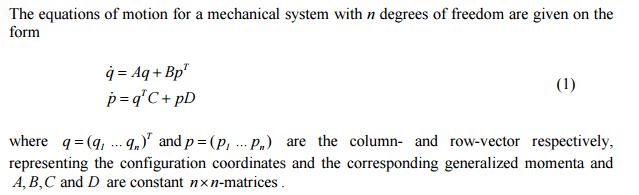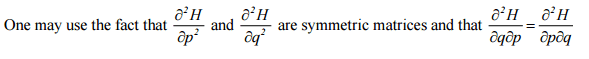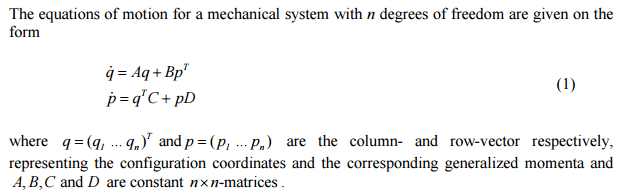# What is the derivative of a matrix transpose?

• I
• dynamicskillingme

#### dynamicskillingme

Hi! As the title says, what is the derivative of a matrix transpose?I am attempting to take the derivative of \dot{q} and \dot{p} with respect to p and q (on each one).

AlsoHi! As the title says, what is the derivative of a matrix transpose?I am attempting to take the derivative of \dot{q} and \dot{p} with respect to p and q (on each one).